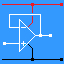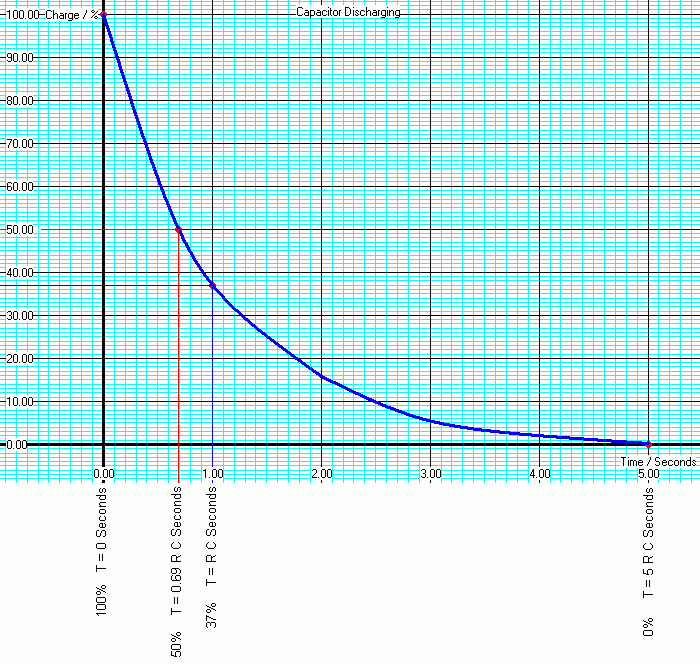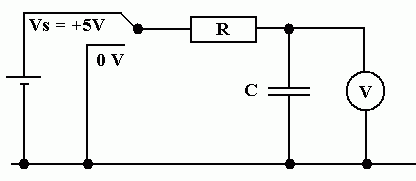RANDOM PAGE

SITE SEARCH

LOG
IN

HELP

# RC Timing Circuits

This is the AQA version closing after June 2019. Visit the the version for Eduqas instead.

## Time Constant: T = R C

• Capacitors can be used, with a resistor, for timing.
• The 555 timer relies on this.

The time constant calculations below are needed for designing timing circuits.

• T is the time in seconds.
• R is the resistor value in Ohms.
• C is the capacitor value in Farads.

Here is a timing circuit. Click the switch the start charging or discharging ...

Advanced Science: In this circuit, when the switch is flipped, the voltage across the capacitor changes quite fast and the current flowing is larger. As the capacitor approaches full charge (or discharge) the voltage changes more slowly and the current is smaller. The current is proportional to the rate of change of voltage. This is completely unlike resistor behaviour where the current is proportional to the voltage.

## RC Timing Calculations

### ChargingR = 1000000 Ohms. C = 1 Microfarad. R C = 1 Second. 0% T = 0 Seconds 50% T = 0.69 R C Seconds 63% T = R C Seconds 66.6% T = 1.1 R C Seconds (555 Monostable) 100% T = 5 R C Seconds

### DischargingR = 1000000 Ohms. C = 1 Microfarad. R C = 1 Second. 100% T = 0 Seconds 50% T = 0.69 R C Seconds 37% T = R C Seconds 0% T = 5 R C Seconds

## Experiment

The aim is to measure the voltage across a charging capacitor at regular time intervals. Once the capacitor is fully charged, repeat the measurements while it is discharging. These results should be plotted on a graph. The graph should then be marked up with the key positions listed above.

• R = 47kΩ
• Charge for 10 minutes. Connect the resistor to the 5 Volt supply.
• Discharge for 10 minutes. Connect the resistor to the 0 Volt supply.
• Don't turn off the stopwatch at half time!• Calculate the time constant T = RC
• Note that capacitors are not usually manufactured with great accuracy and DC leakage may be noticeable when you take your measurements.

## Plot and Label the Graph

Charging ...

• Mark the graph at the 50% mark after 0.69RC seconds
• Mark the graph at the 63% mark after RC seconds
• Mark the graph at the 66.6% mark after 1.1RC seconds
• Mark the graph at the 100% mark after 5RC seconds

Discharging

• Mark the graph at the 50% mark after 0.69RC seconds
• Mark the graph at the 37% mark after RC seconds
• Mark the graph at the 0% mark after 5RC seconds

### Leakage

• Estimate the leakage resistance of the capacitor. (This may well not be constant.)

## Picoscope Traces for a Faster Timing Circuit

• R = 1 kΩ
• C = 0.47 µFFor the Falstad Circuit Simulation, CTRL+Click DC RC Timing Circuit
In options, check European Resistors and uncheck Conventional Current.
Click the switch to charge and discharge the capacitor

Alternatively view RC_Timing.txt.
Save or copy the text on the web page. Import the saved or copied text into the Falstad simulator.

Here is the new HTML5 Simulator Site.

## A2 ONLY: Reactance of a Capacitor

This is the AC equivalent of resistance.

Xc = 1 / ( 2 p F C )

• Xc is the reactance measured in Ohms.
• F is the frequency.
• C is the capacitance.
• Xc is inversely proportional to F (As F increases, Xc decreases).
• Xc is inversely proportional to C (As C increases, Xc decreases).

reviseOmatic V3     Contacts, ©, Cookies, Data Protection and Disclaimers Hosted at linode.com, London